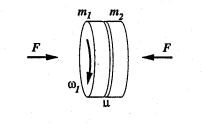# Torque of a rotating wheel on a static wheel

Jenny Physics

## Homework Statement

A uniform cylindrical wheel of mass ##m_{1}## and radius ##R_{1}## rotates with angular velocity ##\omega_{1}##. It lies a certain distance (along the same axis) from a static wheel of radius ##R_{2}## and mass ##m_{2}##. The wheels are then pushed against each other with a constant force ##F## uniformly distributed across the wheel's face. There is friction ##\mu## between the wheels.When the wheels first touch what is the magnitude of the torque that wheel 1 exerts on wheel 2 via friction?

## Homework Equations

Split wheel 1 into infinitesimal concentric rings of radius ##r## and width ##dr## and find the torque exerted by one of these then integrate to find the total torque.

## The Attempt at a Solution

I probably should use ##d\tau=dI \alpha## where ##dI## is the moment of inertia due to the cylindrical ring. But since ##\omega_{1}=const## it would seem that ##\alpha=\dot{\omega}_{1}=0##?

Homework Helper

## Homework Statement

A uniform cylindrical wheel of mass ##m_{1}## and radius ##R_{1}## rotates with angular velocity ##\omega_{1}##. It lies a certain distance (along the same axis) from a static wheel of radius ##R_{2}## and mass ##m_{2}##. The wheels are then pushed against each other with a constant force ##F## uniformly distributed across the wheel's face. There is friction ##\mu## between the wheels.
View attachment 232622
When the wheels first touch what is the magnitude of the torque that wheel 1 exerts on wheel 2 via friction?

## Homework Equations

Split wheel 1 into infinitesimal concentric rings of radius ##r## and width ##dr## and find the torque exerted by one of these then integrate to find the total torque.

## The Attempt at a Solution

I probably should use ##d\tau=dI \alpha## where ##dI## is the moment of inertia due to the cylindrical ring. But since ##\omega_{1}=const## it would seem that ##\alpha=\dot{\omega}_{1}=0##?

You are interested in torque exerted when they touch. The normal force pushing them together will cause a tangential force through friction that will exert the torque. Thing about using ##\tau = r \times F##.

•Jenny Physics
Jenny Physics
You are interested in torque exerted when they touch. The normal force pushing them together will cause a tangential force through friction that will exert the torque. Thing about using ##\tau = r \times F##.
Using ##d\tau=r\frac{F}{\pi R_{1}^{2}}\pi rdr## and then ##\tau=\int_{0}^{R_{1}}d\tau##?

Homework Helper
Gold Member
2021 Award
Using ##d\tau=r\frac{F}{\pi R_{1}^{2}}\pi rdr## and then ##\tau=\int_{0}^{R_{1}}d\tau##?
Close. The surface of a ring of thickness ##dr## and radius ##r## is ##dA=2\pi r dr##.

•Jenny Physics
Homework Helper
Close. The surface of a ring of thickness ##dr## and radius ##r## is ##dA=2\pi r dr##.

And ##F## is the normal force. The force acting against the rotation is the frictional force. There is a ##\mu## involved!

•Jenny Physics
Jenny Physics
Close. The surface of a ring of thickness ##dr## and radius ##r## is ##dA=2\pi r dr##.
Then ##d\tau=r\frac{F}{\pi R_{1}^{2}}2\pi rdr=2\frac{F}{R^{2}_{1}}r^{2}dr## and then ##\tau=\int_{0}^{R_{1}}d\tau=\frac{2}{3}R_{1}F##? The torque will be tangential to the surface and counterclockwise?

Jenny Physics
And ##F## is the normal force. The force acting against the rotation is the frictional force. There is a ##\mu## involved!
The friction force torque will then be ##\tau=-\frac{2\mu}{3}R_{1}F_{1}## so that the total torque will be ##\tau=\frac{2}{3}R_{1}F_{1}(1-\mu)## in the same direction as ##\omega_{1}##?

Homework Helper
Gold Member
2021 Award
The friction force torque will then be ##\tau=-\frac{2\mu}{3}R_{1}F_{1}## so that the total torque will be ##\tau=\frac{2}{3}R_{1}F_{1}(1-\mu)## in the same direction as ##\omega_{1}##?
Yes. Since the angular speed of the wheel is increasing, the torque on it and its angular velocity must be in the same direction.

Where does ##(1-\mu)## come from? What happens when ##\mu = 1##? Check your integral.

Jenny Physics
Yes. Since the angular speed of the wheel is increasing, the torque on it and its angular velocity must be in the same direction.

Where does ##(1-\mu)## come from? What happens when ##\mu = 1##? Check your integral.
Not following. The ##(1-\mu)## comes from the torque of ##F## and the opposite torque of the friction force which is ##\mu F##. Am I missing ##2\pi## in the integral?

Homework Helper
Gold Member
2021 Award
Newton's 3rd law says that to every action there is an equal and opposite reaction. In other words, you change the sign but not the magnitude. This applies to forces and, since torques are generated by forces, it applies to torques as well.

•Jenny Physics
Homework Helper
Not following. The ##(1-\mu)## comes from the torque of ##F## and the opposite torque of the friction force which is ##\mu F##. Am I missing ##2\pi## in the integral?

##F## doesn't produce any net torque, contributions from opposite sides of the wheel cancel. Only the frictional force produces a net torque.

•Jenny Physics
Jenny Physics
##F## doesn't produce any net torque, contributions from opposite sides of the wheel cancel. Only the frictional force produces a net torque.
The torque will then be ##\frac{4\pi}{3}R_{1}\mu F##?

Homework Helper
The torque will then be ##\frac{4\pi}{3}R_{1}\mu F##?

Where did the '4' come from?

Jenny Physics
Where did the '4' come from?
Kuruman mentioned my integral was missing something I thought I was missing a ##2\pi##. Perhaps he meant the factor ##1-\mu## should just be ##\mu##?

Homework Helper
Kuruman mentioned my integral was missing something I thought I was missing a ##2\pi##. Perhaps he meant the factor ##1-\mu## should just be ##\mu##?

You are throwing factors in because you are guessing. Go over it from the beginning and show what YOU think the result should be.

Jenny Physics
You are throwing factors in because you are guessing. Go over it from the beginning and show what YOU think the result should be.
I think it should be ##\frac{2}{3}R_{1}\mu F##

Homework Helper
I think it should be ##\frac{2}{3}R_{1}\mu F##

And I think that's correct.

•Jenny Physics
•